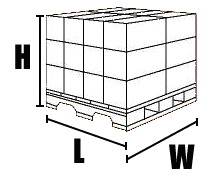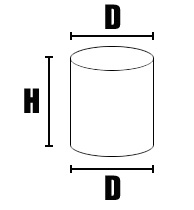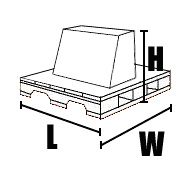Sign Up | Log In# Density

Entering the correct density of your materials on your bill of lading will help ensure that your shipment is correctly rated; most classification changes occur because the shipment density was not calculated correctly. Having the correct density helps to avoid additional charges for research and recalculation.

Refer to the information below for formulas of how to calculate density.

How to calculate density (please use U.S. inches and
pounds):

 1 Determine the weight of your goods in pounds. 2 Measure your shipment's length, width and height in inches. 3 Multiply the length by the width by the height in inches. 4 Divide the total in Step 2 by 1728 to convert cubic inches to cubic feet. 5 Divide your shipment's weight by the total in Step 4.

How to calculate dimensional weight (please use U.S.
inches):

 1 Multiply your shipment's length by its width by its height in inches. 2 Divide the total in Step 1 by 194. This is your dimensional weight.

Formulas:
 Density=Weight/Cubic Feet Cubic feet for crates and odd-size shipments = (H" x W" x L")/1728 Cubic feet for cylinders=(H" x D" x D")/1728 Dimensional Weight=L" x W" x H"/194
Where:
 H=height     W=width     L=length D=diameter     "=inches
Conversions from metric to U.S. measurements:
1 kilo = 2.205 lb
1 cm = .3937 in

Refer to the images below for examples of how to determine your shipment's volume.